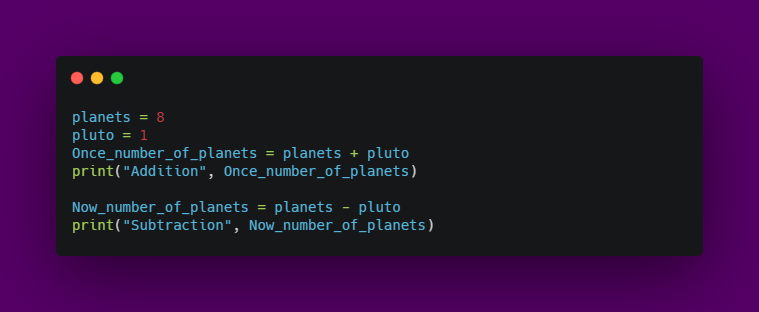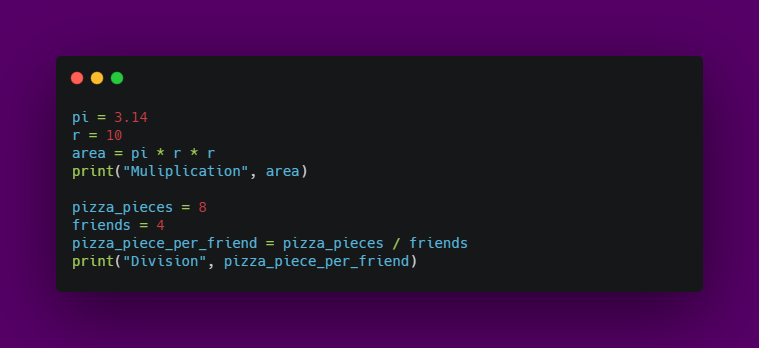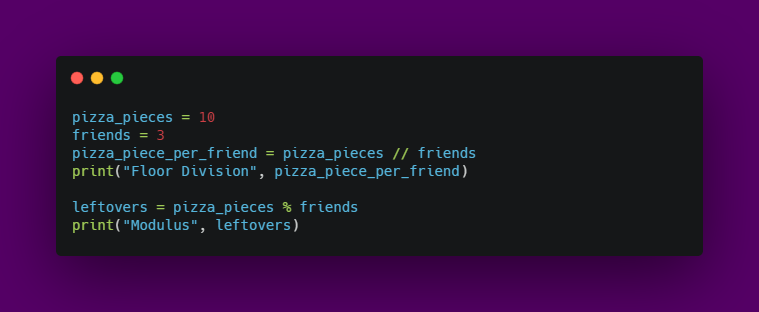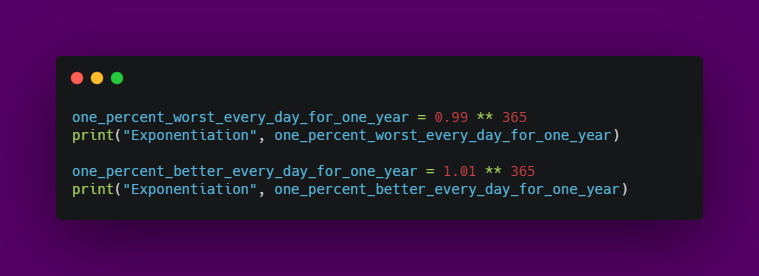Arithmetic operators in python

Operators are used to performing operations on any given data stored inside variables.

And arithmetic operators makes mathematical operations possible on operands in a program.

I know! I know! This is a basic concept! But let’s make it fun!Output:

Subtraction 8

Now, Multiplication and DivisionOutput:

Multiplication 314.0
Division 2.0

Special arithmetic operators

• Floor division - //: rounds of the result to the nearest whole number.
• Modulus - %: produces the remainder of the numbersOutput:
Floor Division 3
Modulus 1

• Exponentiation - ** : produces the power of given numbersOutput:
Exponentiation 0.025517964452291125
Exponentiation 37.78343433288728

Code along and have fun ;)Aswin Barath

My name is Aswin Barath. I’m a technical blogger and budding software engineer